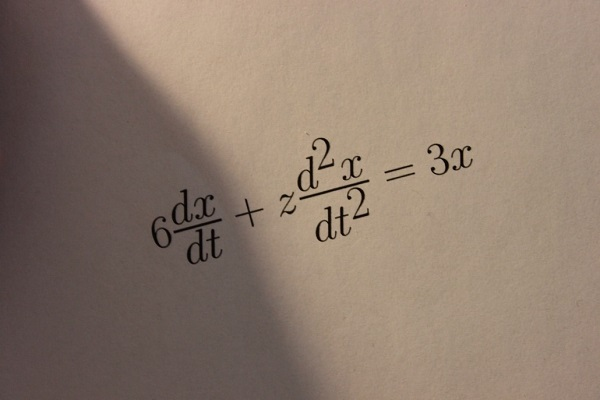Sale!

# LATEX Generation from image of equations

15

## Description

This article describes a system that takes a photograph of a printed equation and produces a LATEX code representation. The process uses adaptive thresholding with mean filtering, morphological edge smoothing, and the Hough transform for image binarization and skew correction.

The project uses Hu invariant moments and circular topology to match characters against a database of characters. The algorithm then assembles the appropriate LATEX code from the detected characters.

This system is able to detect 93.6% of characters in ideal images and 86.2% of characters in real-world photographs when combining two different skew methods (74%-79%).Inputs:
Photographs of printed mathematical expressions

Outputs:
LaTeX code for the mathematical expression

MATLAB code of motion equations for vertical balancing treaded rover

flow rate calculation in the microfluidic channels using the rectangular channel flow equations

## Reviews

There are no reviews yet.

SKU: P2018F208 Category: